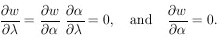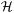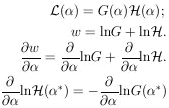### 10. UNIQUENESS OF MAXIMUM-LIKELIHOOD SOLUTION

Usually it is a matter of taste what physical quantity is chosen as. For example, in a lifetime experiment some workers would solve for the lifetime,*, while others would solve for*, where= 1/. Some workers prefer to use momentum, and others energy, etc. Consider the case of two related physical parametersand. The maximum-likelihood solution foris obtained from the equation ðw / ð= 0. The maximum-likelihood solution foris obtained from ðw / ð= 0. But then we haveThus the condition for the maximum-likelihood solution is unique and independent of the arbitrariness involved in choice of physical parameter. A lifetime result* would be related to the solution* by* = 1/*.

The basic shortcoming of the maximum-likelihood method is what to do about the prior probability of. If the prior probability ofis G() and the likelihood function obtained for the experiment alone is(), then the joint likelihood function isgive the maximum-likelihood solution. In the absence of any prior knowledge the term on the right-hand side is zero. In other words, the standard procedure in the absence of any prior information is to use a prior distribution in which all values ofare equally probable. Strictly speaking, it is impossible to know a "true" G(), because it in turn must depend on its own prior probability. However, the above equation is useful when G() is the combined likelihood function of all previous experiments and() is the likelihood function of the experiment under consideration.

There is a class of problems in which one wishes to determine an unknown distribution in, G(), rather than a single value. For example, one may wish to determine the momentum distribution of cosmic ray muons. Here one observeswhere(; x) is known from the nature of the experiment and G() is the function to be determined. This type of problem is discussed in Reference 5.